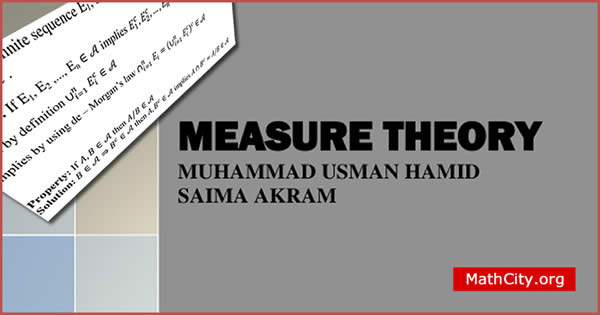# Measure Theory by M Usman Hamid & Saima AkramThe study of measures on sets is the focus of the mathematical field known as measure theory. A measure is a function that gives specific subsets of a given set a non-negative real integer, indicating their size inferentially. The concept of measure is a formalisation and generalisation of common concepts like mass and event probability as well as geometrical measurements (length, area, and volume).

Modern analysis and probability theory are built on a basis provided by measure theory. It is used in many branches of mathematics, such as partial differential equations, probability theory, ergodic theory, geometric measure theory, and functional and harmonic analysis.

These 127 pages of notes, which cover a wide range of measure theory subjects, are provided on this website. It begins with algebra and sigma algebra and ends with the measure product. One can learn important topics of the measure theory from these notes. So we can say that these are the best notes of the measure theory. The main aim of this course is to develop a more satisfactory theory of integration to overcome the drawbacks menioned in the notes by Muhammad Usman Hamid

The Riemann integral, dealt with in calculus courses, is well suited for computations but less suited for dealing with limit processes. In this course we will introduce the so called Lebesgue integral, which keeps the advantages of the Riemann integral and eliminates its drawbacks by Saima Akram

• Name: Measure Theory
• Author: Muhammad Usman Hamid & Saima Akram
• Pages: 127 pages
• Format: PDF
• Size: 2.02 MB
* Algebras and Sigma-Algebras
• Measures, Outer Measures
• Lebesgue Measure
• Measurable sets and Lebesgue measure
• A non-measurable set
• Completeness and Regularity
• Functions and Integrals
• Measurable Functions
• Properties That Hold Almost Everywhere
• Lebesgue integration: the Lebesgue integral of a non-negative function
• The general Lebesgue integral;
• General measure and integration measure spaces
• The Integral
• Limit Theorems
• The Riemann Integral
• Measurable Functions Again
• Complex-Valued Functions, and
• Image Measures, Convergence
• Modes of Convergence
• Definition and Properties Signed measures
• Outer measure and measurability
• The extension theorem
• The Lebesgue Stieltjes integral
• Product measures

• notes/measure-thoery-muhsa